Question

# Exam Name MULTIPLE CHOICE. Choose the one alternative that best completes the statement or answers the...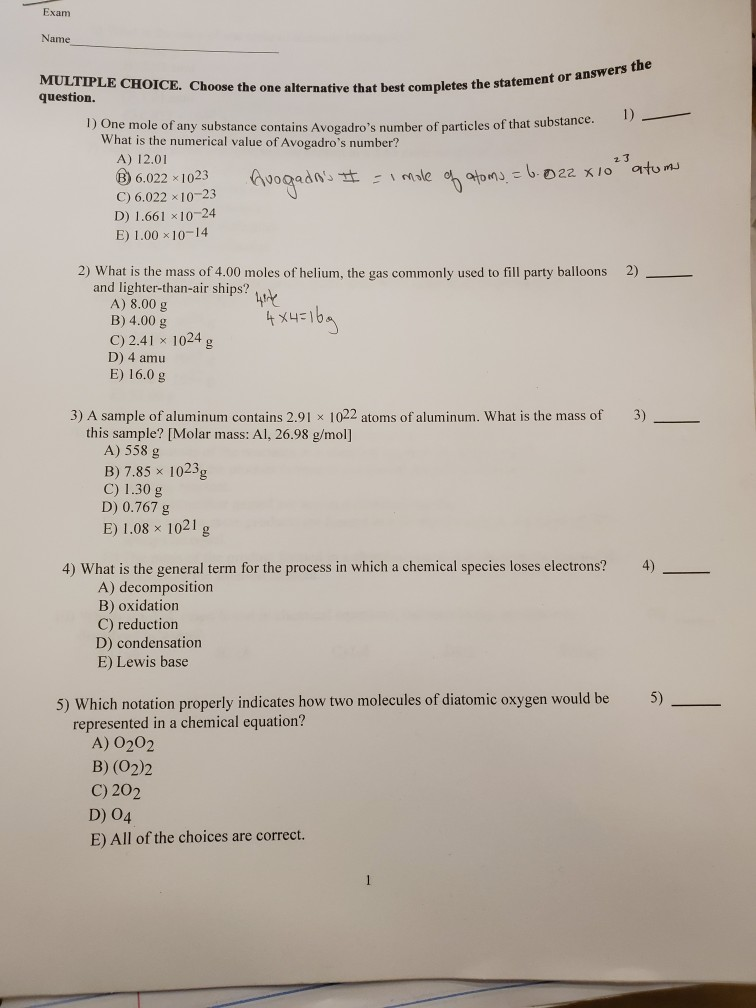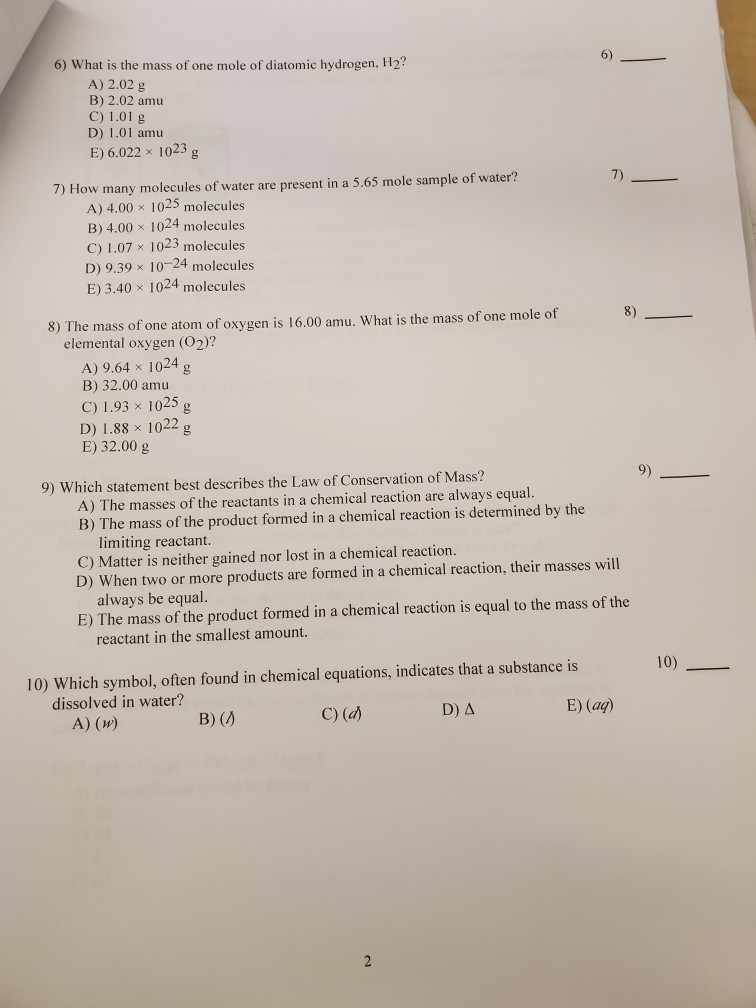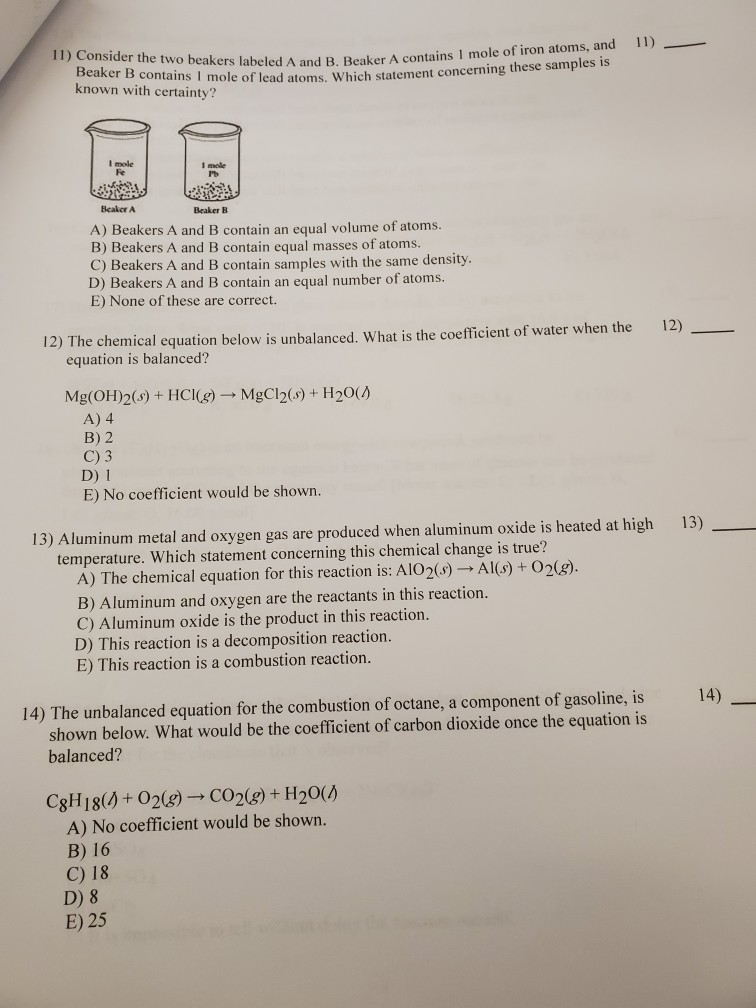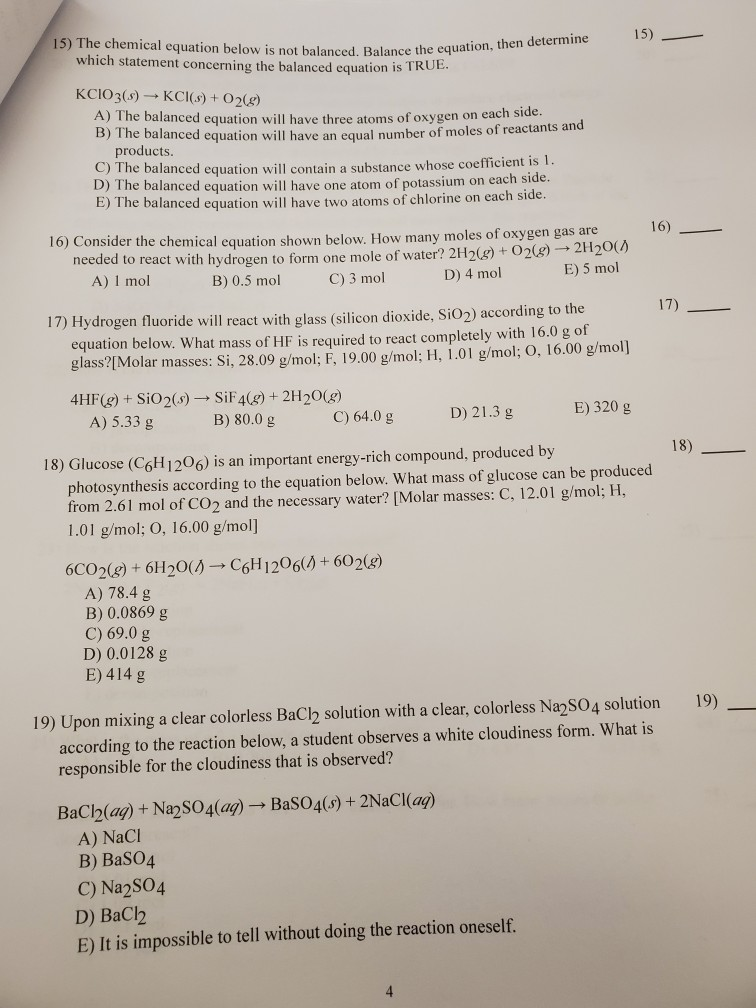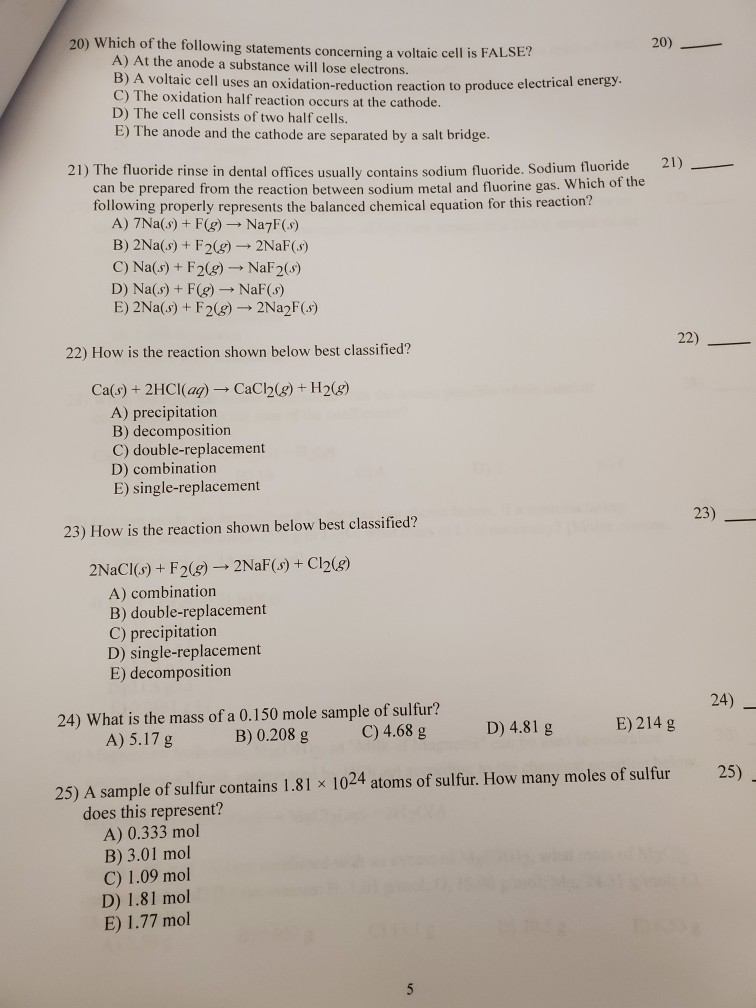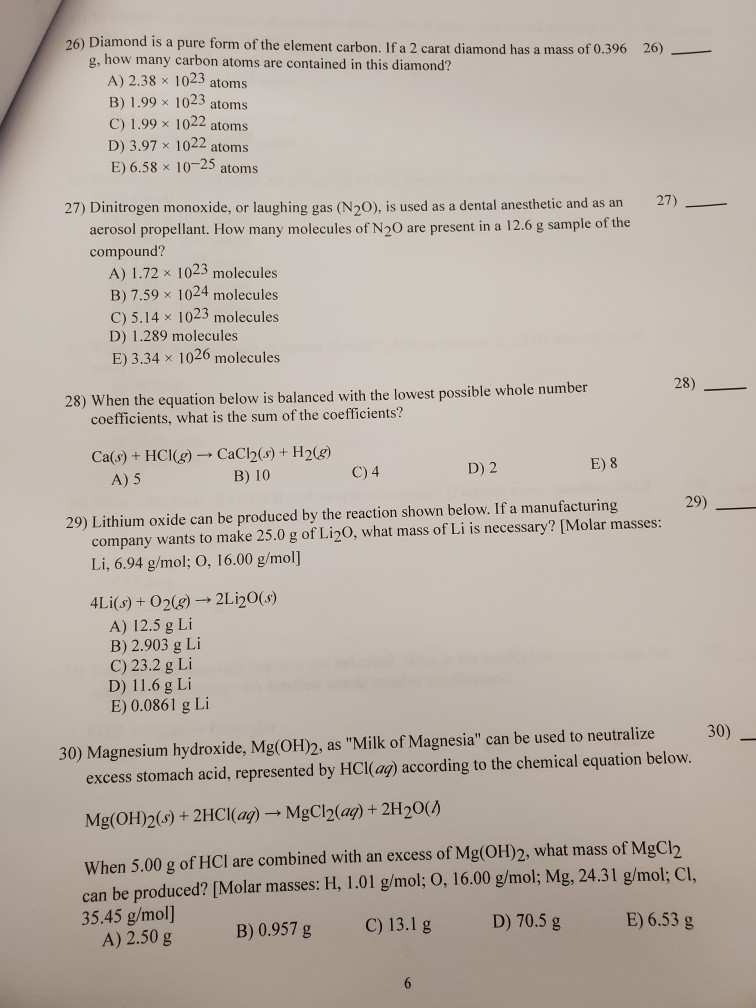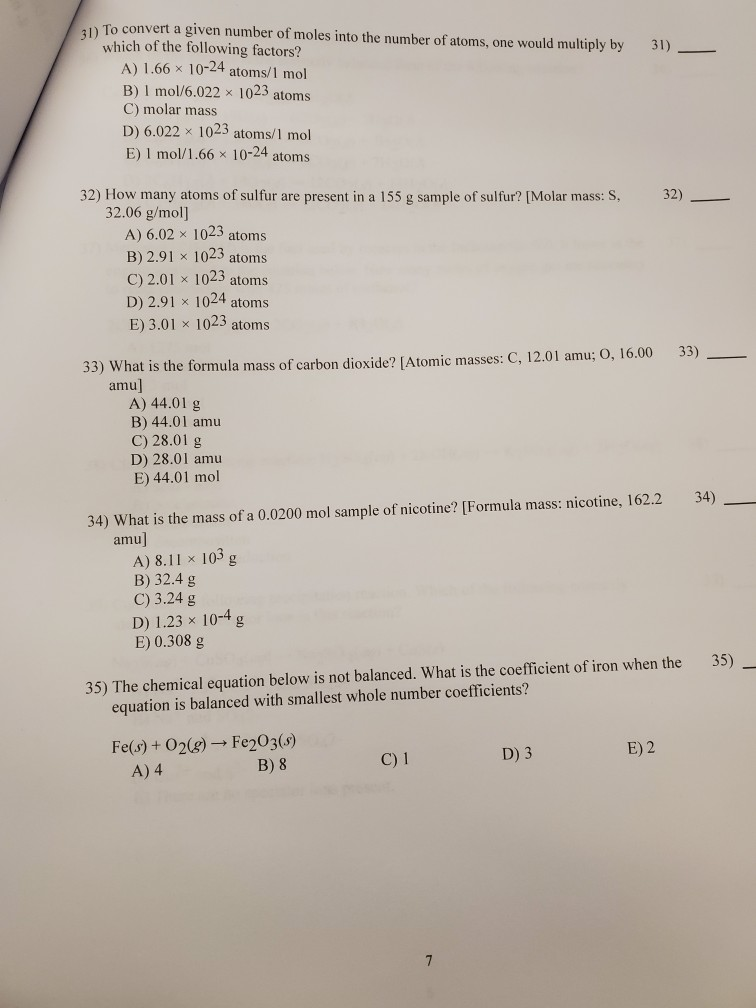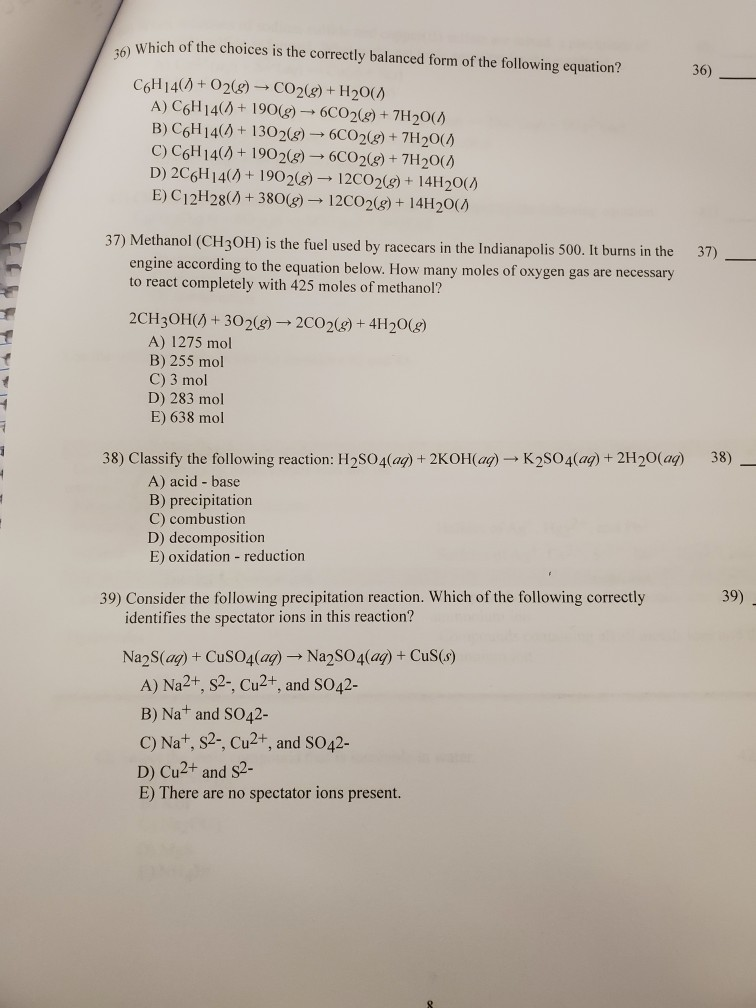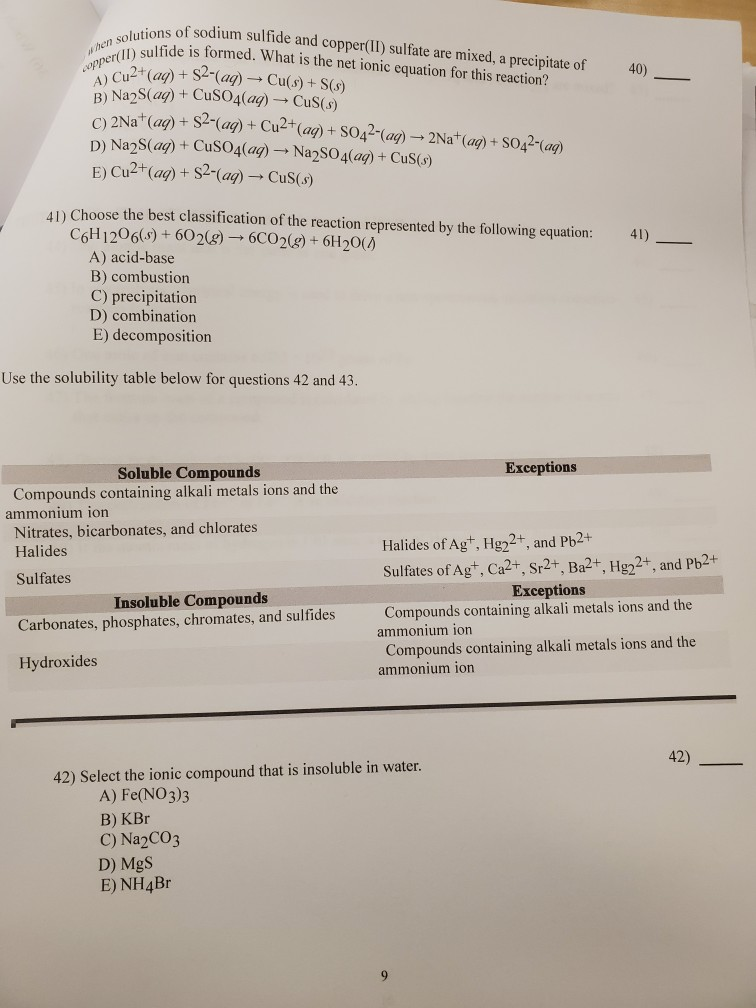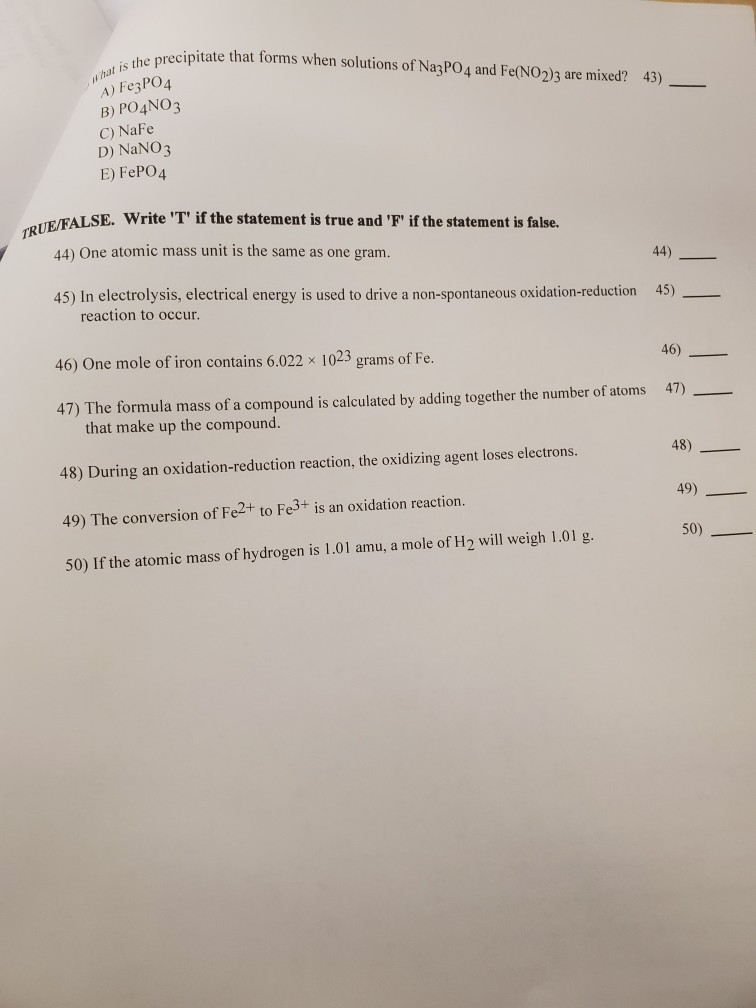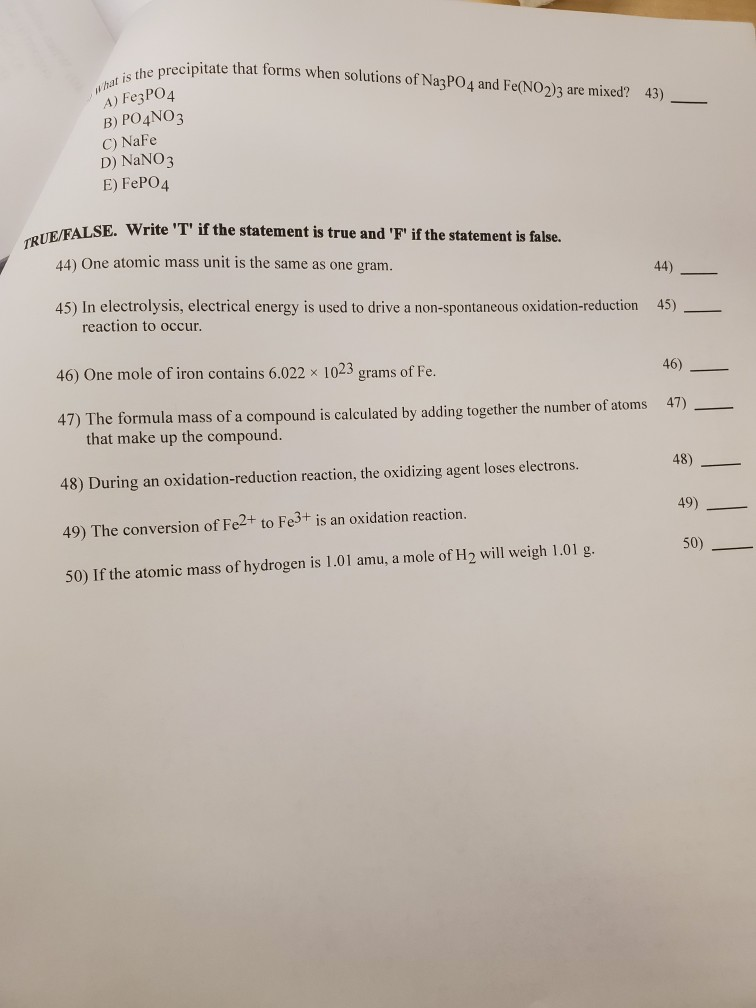Exam Name MULTIPLE CHOICE. Choose the one alternative that best completes the statement or answers the question. 1) 1) One mole of any substance contains Avogadro's number of particles of that substance. What is the numerical value of Avogadro's number? A) 12.01 23 Avagrans t Male aton 6022 x1o atums B) 6.022 x 1023 C) 6.022 x 10-23 D) 1.661 x10-24 E) 1.00 x10-14 2) What is the mass of 4.00 moles of helium, the gas commonly used to fill party balloons and lighter-than-air ships? A) 8.00 g B) 4.00 g C) 2.41 x 1024 g D) 4 amu E) 16.0 g 2) 4 3) A sample of aluminum contains 2.91 x 1022 atoms of aluminum. What is the mass of this sample? [Molar mass: Al, 26.98 g/mol] A) 558 g B) 7.85 x 1023g C) 1.30 g D) 0.767 g E) 1.08 x 1021 g 3) 4) What is the general term for the process in which a chemical species loses electrons? A) decomposition B) oxidation 4) C) reduction D) condensation E) Lewis base 5) Which notation properly indicates how two molecules of diatomic oxygen would be represented in a chemical equation? A) 0202 5) B) (02)2 C) 202 D) 04 E) All of the choices are correct. 1
6) What is the mass of one mole of diatomic hydrogen, H2? 6) A) 2.02 g B) 2.02 amu C) 1.01 g D) 1.01 amu E) 6.022 x 1023 g 7) How many molecules of water are present in a 5.65 mole sample of water? A) 4.00 x 1025 molecules B) 4.00x 1024 molecules C) 1.07 x 1023 molecules D) 9.39 x 10-24 molecules E) 3.40 x 1024 molecules 7) 8) The mass of one atom of oxygen is 16.00 amu. What is the mass of one mole of elemental oxygen (O2)? 8) A) 9.64 x 1024 B) 32.00 amu C) 1.93 x 1025 g D) 1.88 x 1022 E) 32.00 g g 9) Which statement best describes the Law of Conservation of Mass? A) The masses of the reactants in a chemical reaction are always equal. B) The mass of the product formed in a chemical reaction is determined by the limiting reactant. C) Matter is neither gained nor lost in a chemical reaction. D) When two or more products are formed in a chemical reaction, their masses will always be equal. E) The mass of the product formed in a chemical reaction is equal to the mass of the reactant in the smallest amount. 10) Which symbol, often found in chemical equations, indicates that a substance is dissolved in water? A) (w) 10) E) (aq) D) A C) (d) B) (A 2
11) Consider the two beakers labeled A and B. Beaker A contains I mole of iron atoms, and 11) Beaker B contains 1 mole of lead atoms. Which statement concerning these samples is known with certainty? I mole Fe mole Beaker A Beaker B A) Beakers A and B contain an equal volume of atoms B) Beakers A and B contain equal masses of atoms. C) Beakers A and B contain samples with the same density. D) Beakers A and B contain an equal number of atoms. E) None of these are correct. 12) The chemical equation below is unbalanced. What is the coefficient of water when the equation is balanced? 12) Mg(OH)2(s)+HCl (g)MgCl2(s)+H20() A) 4 B) 2 С) 3 D) 1 E) No coefficient would be shown. 13) Aluminum metal and oxygen gas are produced when aluminum oxide is heated at high temperature. Which statement concerning this chemical change is true? A) The chemical equation for this reaction is: AIO2(s)Al(s) + O2(g) 13) B) Aluminum and oxygen are the reactants in this reaction. C) Aluminum oxide is the product in this reaction. D) This reaction is a decomposition reaction. E) This reaction is a combustion reaction. 14) 14) The unbalanced equation for the combustion of octane, a component of gasoline, is shown below. What would be the coefficient of carbon dioxide once the equation is balanced? C8H18()+02(g)CO2(g) + H20( A) No coefficient would be shown. B) 16 C) 18 D) 8 E) 25
We were unable to transcribe this image
20) Which of the following statements concerning a voltaic cell is FALSE? 20) A) At the anode a substance will lose electrons. B) A voltaic cell uses an oxidation-reduction reaction to produce electrical energy C) The oxidation half reaction occurs at the cathode. D) The cell consists of two half cells. E) The anode and the cathode are separated by a salt bridge. 21) 21) The fluoride rinse in dental offices usually contains sodium fluoride. Sodium fluoride can be prepared from the reaction between sodium metal and fluorine gas. Which of the following properly represents the balanced chemical equation for this reaction? A) 7Na(s)+F(g)Na7F(s) B) 2Na(s)+F2(g)- 2NaF(s) C) Na(s)+F2(g)NaF 2(s) D) Na(s)F(g)NaF(s) E) 2Na(s) + F2(g)2Na2F(s) 22) 22) How is the reaction shown below best classified? Ca(s)+ 2HCI(aq) -CaC2(g) + H2(g) A) precipitation B) decomposition C) double-replacement D) combination E) single-replacement 23) 23) How is the reaction shown below best classified? 2NaCl(s)F2(g)2NaF(s) + Cl2(g) A) combination B) double-replacement C) precipitation D) single-replacement E) decomposition 24) 24) What is the mass of a 0.150 mole sample of sulfur? B) 0.208 g E) 214 g D) 4.81 g C) 4.68 g A) 5.17 g 25) A sample of sulfur contains 1.81 x 1024 atoms of sulfur. How many moles of sulfur does this represent? A) 0.333 mol B) 3.01 mol C) 1.09 mo D) 1.81 mol E) 1.77 mol 25) 5
26) Diamond is a pure form of the element carbon. If a 2 carat diamond has a mass of 0.396 g, how many carbon atoms are contained in this diamond? 26) A) 2.38 x 1023 atoms B) 1.99 x 1023 atoms C) 1.99 x 1022 atoms D) 3.97 x 1022 atoms E) 6.58 x 10-25 atoms 27) Dinitrogen monoxide, or laughing gas (N2O), is used as a dental anesthetic and as an aerosol propellant. How many molecules of N20 are present in a 12.6 g sample of the 27) compound? A) 1.72 x 1023 molecules B) 7.59 x 1024 molecules C) 5.14 x 1023 molecules D) 1.289 molecules E) 3.34 x 1026 molecules 28) 28) When the equation below is balanced with the lowest possible whole number coefficients, what is the sum of the coefficients? Ca(s)+ HCl(g) CaC2(s) + H2(g) B) 10 E) 8 D) 2 C) 4 A) 5 29) 29) Lithium oxide can be produced by the reaction shown below. If a manufacturing company wants to make 25.0 g of Li2O, what mass of Li is necessary? [Molar masses: Li, 6.94 g/mol; O, 16.00 g/mol] 4Li(s)+ 02(g)-2Li2O(s) A) 12.5 g Li B) 2.903 g Li C) 23.2 g Li D) 11.6 g Li E) 0.0861 g Li 30) Magnesium hydroxide, Mg(OH)2, as "Milk of Magnesia" can be used to neutralize excess stomach acid, represented by HCl(ag) according to the chemical equation below. 30) Mg(OH)2(s)+ 2HC| (aq)MgCl2(ag)+ 2H20() When 5.00 g of HCl are combined with an excess of Mg(OH)2, what mass of MgCl2 can be produced? [Molar masses: H, 1.01 g/mol; O, 16.00 g/mol; Mg, 24.31 g/mol; Cl 35.45 g/mol] A) 2.50 g E) 6.53 g D) 70.5 g C) 13.1 g B) 0.957 g
To convert a given number of moles into the number of atoms, one would multiply by which of the following factors? A) 1.66 x 10-24 atoms/1 mol B) I mol/6.022x 1023 atoms C) molar mass 31) D) 6.022 x 1023 atoms/1 mol E) I mol/1.66 x 10-24 atoms 32) How many atoms of sulfur are present in a 155 g sample of sulfur? [Molar mass: S, 32.06 g/mol] A) 6.02 x 1023 atoms B) 2.91 x 1023 atoms C) 2.01 x 1023 atoms D) 2.91 x 1024 atoms E) 3.01 x 1023 atoms 32) 33) 33) What is the formula mass of carbon dioxide? IAtomic masses: C, 12.01 amu; O, 16.00 amu] A) 44.01 g B) 44.01 amu C) 28.01 g D) 28.01 amu E) 44.01 mol 34) What is the mass of a 0.0200 mol sample of nicotine? [Formula mass: nicotine, 162.2 amu] A) 8.11 x 103 g B) 32.4 g C) 3.24 g D) 1.23 x 10-4 g E) 0.308 g 34) 35) The chemical equation below is not balanced. What is the coefficient of iron when the equation is balanced with smallest whole number coefficients? 35) Fe(s)+O2(g) Fe203(s) B) 8 E) 2 D) 3 C) 1 A) 4 7
Which of the choices is the correctly balanced form of the following equation? 36) C6H14(+02(g)CO2()+ H20( A) C6H14(190()6CO2() + 7H20( B) C6H14(130 2(g)6CO2(g)+ 7H20( C) C6H14(190 2(8) + 6CO2(g) + 7H20( D) 2C6H14(1902(g)12CO2(8) + 14H20() E) C12H28(380(g)12CO2(g)+ 14H20( 37) Methanol (CH3OH) is the fuel used by racecars in the Indianapolis 500. It burns in the 37) engine according to the equation below. How many moles of oxygen gas are necessary to react completely with 425 moles of methanol? 2CH30H(A3O2(g)2CO2(g)+ 4H20(g) A) 1275 mol B) 255 mol C) 3 mol D) 283 mol E) 638 mol 38) Classify the following reaction: H2SO4(ag) + 2KOH(ag)K2SO4(ag) + 2H20(ag) 38) 4 A) acid base B) precipitation C) combustion D) decomposition E) oxidation reduction 39) Consider the following precipitation reaction. Which of the following correctly identifies the spectator ions in this reaction? 39) Na2S(aq)+CuS04(ag)Na2SO4(ag)+ CuS(s) A) Na2+, s2-, Cu2+, and SO42- B) Nat and SO42- C) Nat, S2-, Cu2+, and SO42- D) Cu2+ and S2- E) There are no spectator ions present.
when solutions of sodium sulfide and copper(II) sulfate are mixed, a precipitate of copper() sulfide is formed. What is the net ionic equation for this reaction? 40) Cu2 (ag)+S(aq)Cu(s) +S(s) B) Na2S(ag) CuSO4(ag)CuS(s) C)2Na (ag)+ S2-(ag)+ Cu2 (ag) + SO42-(ag)-2Nat (ag)+ SO42-(ag) D) Na2S(ag)+CUSO4(ag)- NaSO 4(ag) + CuS(s) E) Cu2+(ag)+ S2-(ag)-CuS(s) AD Choose the best classification of the reaction represented by the following equation: C6H1206(s)60 2(g) -- 6CO2(g) + 6H20( 41) A) acid-base B) combustion C) precipitation D) combination E) decomposition Use the solubility table below for questions 42 and 43. Еxceptions Soluble Compounds Compounds containing alkali metals ions and the ammonium ion Nitrates, bicarbonates, and chlorates Halides Halides of Agt, Hg22+, and Pb2+ Sulfates of Agt, Ca2+, Sr2+, Ba2+, Hg22+, and Pb2+ Sulfates Exceptions Insoluble Compounds Carbonates, phosphates, chromates, and sulfides Compounds containing alkali metals ions and the ammonium ion Compounds containing alkali metals ions and the ammonium ion Hydroxides 42) 42) Select the ionic compound that is insoluble in water. A) Fe(NO 3)3 B) KBr C) NA2CO3 D) MgS E) NH4BR 9
what is the precipitate that forms when solutions of Na3PO4 and Fe(NO2)3 are mixed? 43) A) Fe3PO4 B) PO4NO3 C) NaFe D) NaNO3 E) FEPO4 TRUE/FALSE. Write 'T' if the statement is true and 'F' if the statement is false. 44) One atomic mass unit is the same as one gram. 44) 45) In electrolysis, electrical energy is used to drive a non-spontaneous oxidation-reduction reaction to occur. 45) 46) One mole of iron contains 6.022 x 102-3 grams of Fe. 46) 47) The formula mass of a compound is calculated by adding together the number of atoms that make up the compound. 47) 48) 48) During an oxidation-reduction reaction, the oxidizing agent loses electrons. 49) 49) The conversion of Fe2+ to Fe3+ is an oxidation reaction 50) 50) If the atomic mass of hydrogen is 1.01 amu, a mole of H2 will weigh 1.01 g.
is the precipitate that forms when solutions of Na3PO4 and Fe(NO2)3 are mixed? 43) A) FE3PO4 B) PO4NO3 C) NaFe D) NaNO3 E) FePO4 TRUE/FALSE. Write 'T' if the statement is true and 'F' if the statement is false. 44) One atomic mass unit is the same as one gram. 44) 45) In electrolysis, electrical energy is used to drive a non-spontaneous oxidation-reduction reaction to occur. 45) 46) One mole of iron contains 6.022 x 1023 grams of Fe. 46) 47) 47) The formula mass of a compound is calculated by adding together the number of atoms that make up the compound. 48) 48) During an oxidation-reduction reaction, the oxidizing agent losses electrons. 49) 49) The conversion of Fe2+ to Fe3+ is an oxidation reaction. 50) 50) If the atomic mass of hydrogen is 1.01 amu, a mole of H2 will weigh 1.01 g.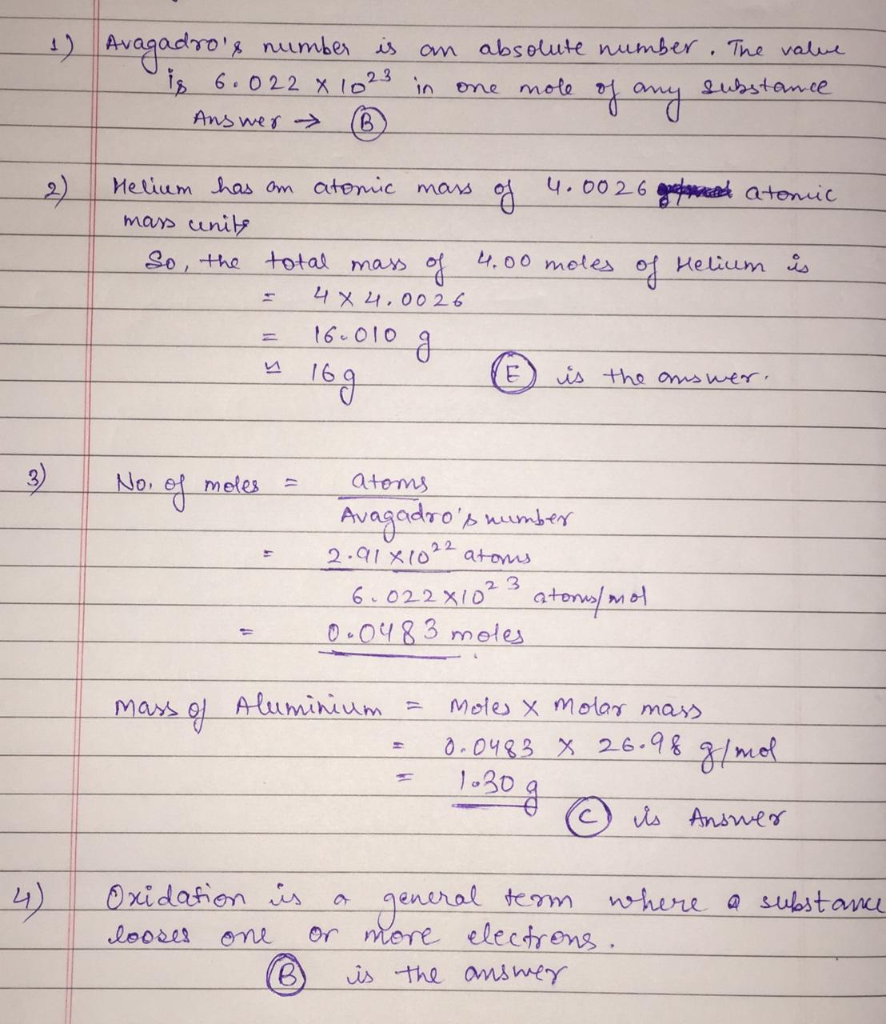#### Earn Coins

Coins can be redeemed for fabulous gifts.

Similar Homework Help Questions
• ### 31). 31) To convert a given number of moles into the number of atoms, one would...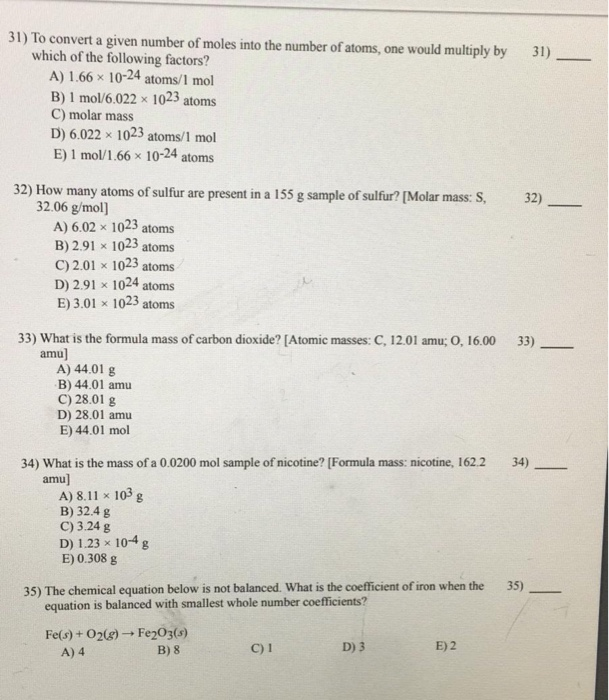31). 31) To convert a given number of moles into the number of atoms, one would multiply by which of the following factors? A) 1.66 x 10-24 atoms/1 mol B) 1 mol/6.022 x 1023 atoms C) molar mass D) 6.022 x 1023 atoms/1 mol E) 1 mol/1.66 x 10-24 atoms 32) 32) How many atoms of sulfur are present in a 155 g sample of sulfur? [Molar mass: S, 32.06 g/mol] A) 6.02 x 1023 atoms B) 2.91 x 1023...

• ### m105%20Makeup%20Exam%20(2).pdf 31) 31) To convert a given number of moles into the number of atoms, one...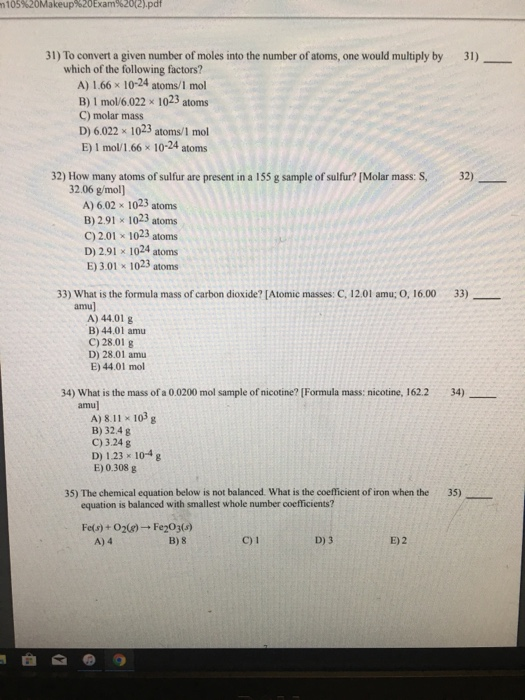m105%20Makeup%20Exam%20(2).pdf 31) 31) To convert a given number of moles into the number of atoms, one would multiply by which of the following factors? A) 1.66 x 10-24 atoms/I mol B) 1 mol/6.022 1023 atoms C) molar mass D) 6.022 * 1023 atoms/1 mol E) 1 mol/1.66 x 10-24 atoms 3 2) 32) How many atoms of sulfur are present in a 155 g sample of sulfur? [Molar mass: S 32.06 g/mol) A) 6.02 x 1023 atoms B) 2.91 x...

• ### 5) How many moles of hydrogen gas are needed to react with oxygen to form one...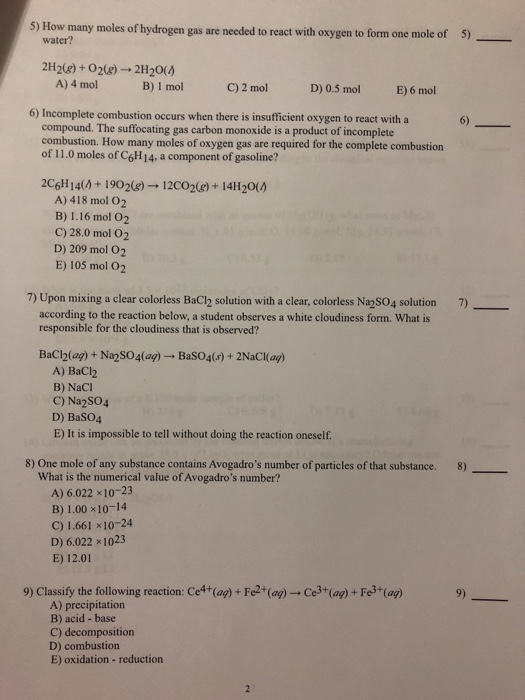5) How many moles of hydrogen gas are needed to react with oxygen to form one mole of 5) water? 2H2(8) + O268) - 2H20(1) A) 4 mol B) 1 mol C) 2 mol D) 0.5 mol E) 6 mol 6) Incomplete combustion occurs when there is insufficient oxygen to react with a compound. The suffocating gas carbon monoxide is a product of incomplete combustion. How many moles of oxygen gas are required for the complete combustion of 11.0 moles...

• ### 5) Choose the best classification of the reaction represented by the following equation: Pb(NO3)2(ay)+CaClg(ay)-PbCl20)+ Ca(NO3)2(a) A)...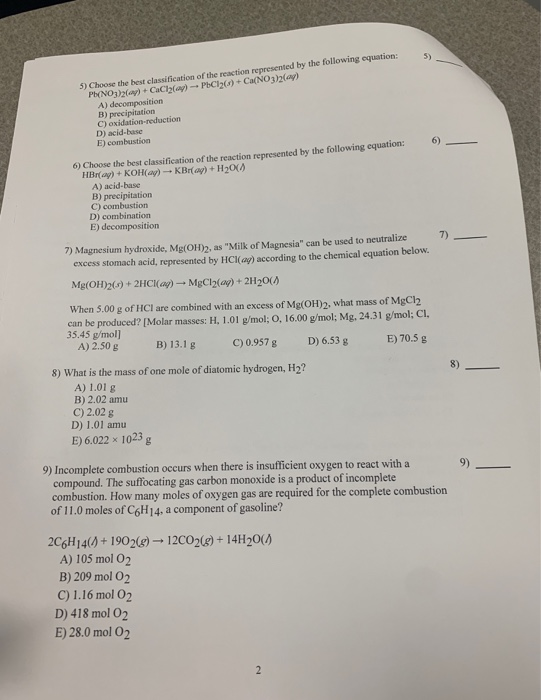5) Choose the best classification of the reaction represented by the following equation: Pb(NO3)2(ay)+CaClg(ay)-PbCl20)+ Ca(NO3)2(a) A) decomposition B) precipitation C) oxidation-reduction D) acid-base E) combustion 5) 6) 6) Choose the best classification of the reaction represented by the following equation: HBr(ay)+ KOH(ag)KBr(ag) + H20( A) acid-base B) precipitation C) combustion D) combination E) decomposition 7) 7) Magnesium hydroxide, Mg(OH)2, as "Milk of Magnesia" can be used to neutralize excess stomach acid, represented by HCl(ag) according to the chemical equation below....

• ### 12) 12) Choose the best classification of the reaction represented by the following equation: 2Fe3 (ag)+...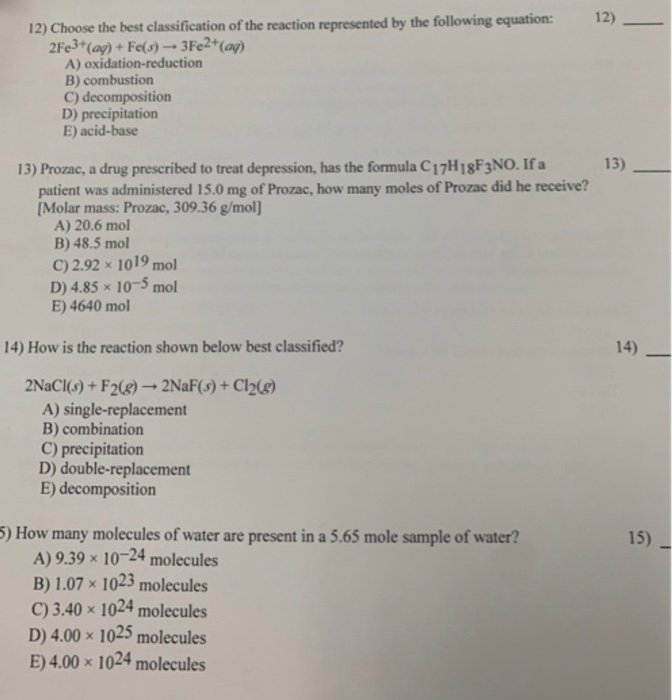12) 12) Choose the best classification of the reaction represented by the following equation: 2Fe3 (ag)+ Fe(s)3Fe2+(ag) A) oxidation-reduction B) combustion C) decomposition D) precipitation E) acid-base 13) 13) Prozac, a drug prescribed to treat depression, has the formula C17H18F3NO. If a patient was administered 15.0 mg of Prozac, how many moles of Prozac did he receive? [Molar mass: Prozac, 309.36 g/mol] A) 20.6 mol B) 48.5 mol C) 2.92 x 1019 mol D) 4.85x 10-5mol E) 4640 mol 14)...

• ### Name MULTIPLE CHOICE. Choose the one als HOICL Choose the one alternative that best completes the...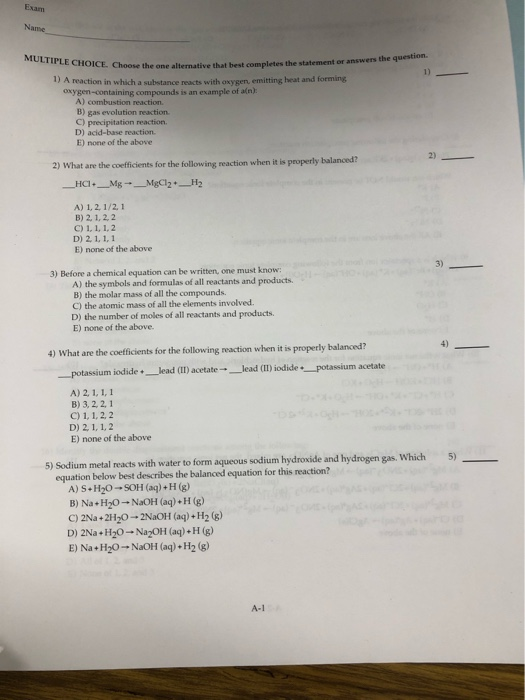Name MULTIPLE CHOICE. Choose the one als HOICL Choose the one alternative that best completes the statement or answer the questio 1) A reaction in which a substance reacts with oxygen, emitting heat and forming oxygen-containing compounds is an example of an): A) combustion reaction B) gas evolution reaction C) precipitation reaction. D) acid-base reaction E) none of the above 2) What are the coefficients for the following reaction when it is properly balanced? _HCl+_Mg _MgCl2+_H2 A) 1.2.1/21 B) 2,1,2,2...

• ### Answer the following. Answers only MULTIPLE CHOICE. Choose the one alternative that best completes the statement...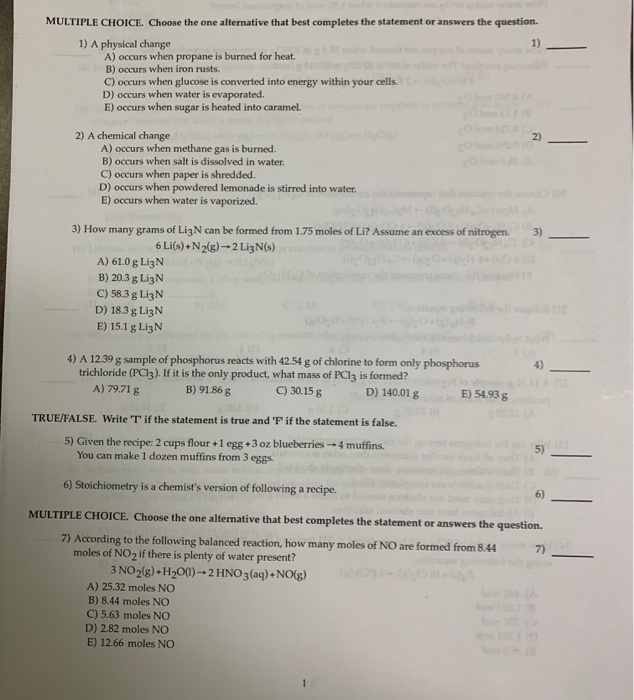Answer the following. Answers only MULTIPLE CHOICE. Choose the one alternative that best completes the statement or answers the question. 1) A physical change A) occurs when propane is burned for heat. B) occurs when iron rusts. C) occurs when glucose is converted into energy within your cells. D) occurs when water is evaporated. E) occurs when sugar is heated into caramel 23 2) A chemical change A) occurs when methane gas is burned. B) occurs when salt is dissolved...

• ### Answer the following. Answers only MULTIPLE CHOICE. Choose the one alternative that best completes the statement...Answer the following. Answers only MULTIPLE CHOICE. Choose the one alternative that best completes the statement or answers the question. 1) A physical change A) occurs when propane is burned for heat. B) occurs when iron rusts. C) occurs when glucose is converted into energy within your cells. D) occurs when water is evaporated. E) occurs when sugar is heated into caramel 23 2) A chemical change A) occurs when methane gas is burned. B) occurs when salt is dissolved...

• ### e precipitate that forms when solutions of Na3PO4 and Fe(NO2)3 are mixed? 43) 2) What is...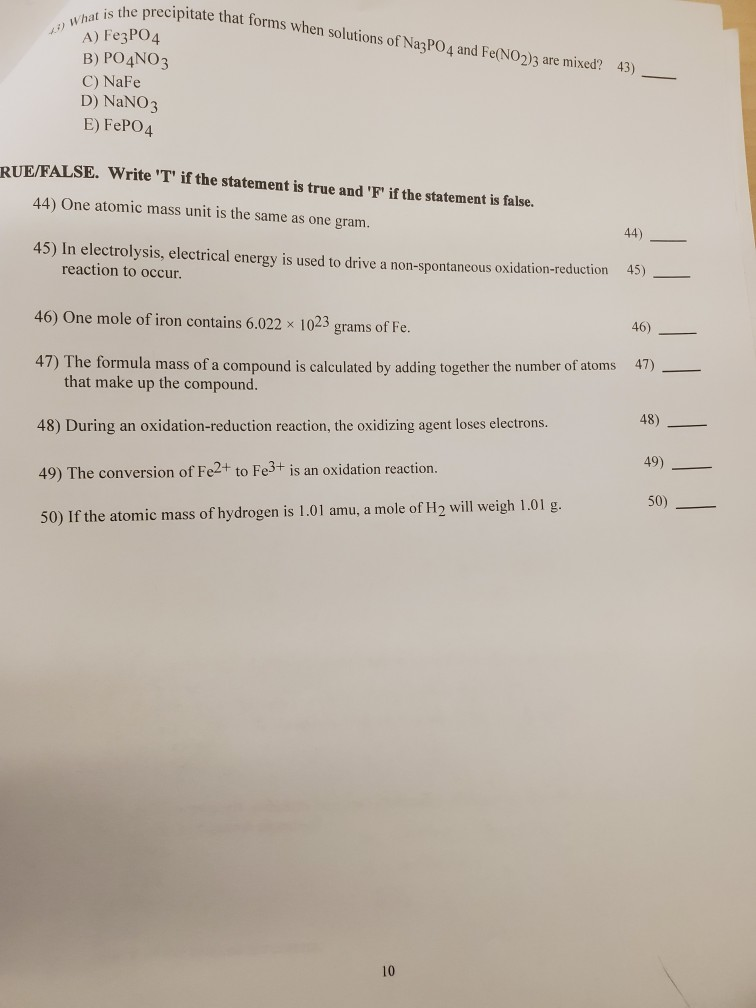e precipitate that forms when solutions of Na3PO4 and Fe(NO2)3 are mixed? 43) 2) What is the precini A) Fe3PO4 B) PO4NO3 C) NaFe D) NaNO3 E) FePO4 RUE/FALSE. Write 'T' if the statement is true and 'F' if the statement is false. 44) One atomic mass unit is the same as one gram. 45) In electrolysis, electrical energy is used to drive a non-spontaneous oxidation-reduction reaction to occur. 45) - 46) One mole of iron contains 6.022 x 1023...

• ### number 9 please MULTIPLE CHOICE. Choose the one alternative that best completes the statement or answen...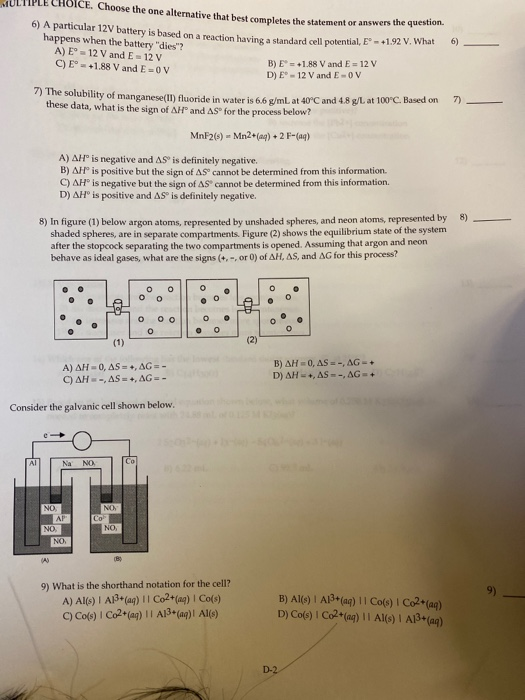number 9 please MULTIPLE CHOICE. Choose the one alternative that best completes the statement or answen the sers the question. 6) A particular 12V battery is bas battery is based on a reaction having a standard cell potential, E 1.92 V. What happens when the battery "dies? A) E° - 12 V and E = 12 V B) E = +1.88 V and E= 12 V C) E = +1.88 V and E=OV D) E-12 V and E-OV ) 1)...